# Polyhedra: Introduction /Double Counting, Dual

Polygons, including the Art Gallery Problem, should have been treated before
Class Activity: The class starts by distributing a set of paper or plastic polygons. The task is to stick these polygons together at common edges to create closed 3-dimensional shapes, so-called polyhedra. Note that edges that are glued together must have the same length, and at each edge just two polygons are glued together.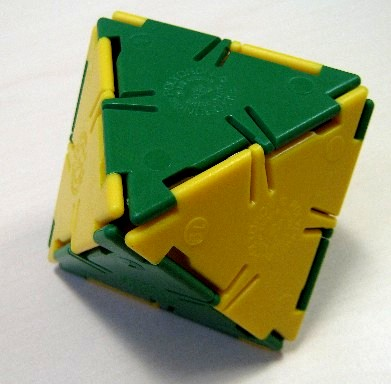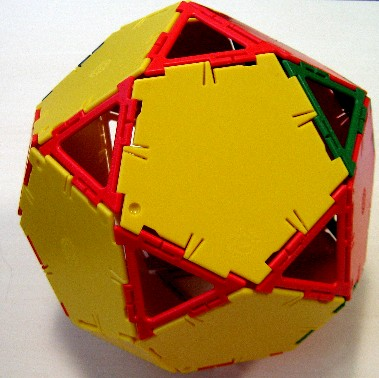Here ares some examples. The empty red triangles in the third example still count as faces.

The flat polygons used as sides of the polyhedron are called the faces. The lines where different polygons are glued together are called the edges. Finally, the vertices are the ends of the lines, the "corners" of a polyhedron. The numbers of faces, edges, and vertices of a polyhedron are usually abbreviated by the letters F, E, and V. Note that the plural of "polyhedron" is "polyhedra".

For the moment, let us define polyhedra aas follows: A polyhedron is a three-dimensional object created by putting flat polygons together such that two polygons are joined by a common edge, and every edge bounds two polygons. Obviously every polyhedron could also be viewed as a graph, since we have vertices---the vertices of the polygons--- that are connected by edges. The degree of a vertex in the polygon is the number of edges it is incident with. It is the same as the degree in the graph-theoretical sense.

For some famous polyhedra, all faces have the same shape---they are regular n-gons for a fixed value of n. This is true for the five Platonic Solids, where all faces are regular polygons of only one shape, and every vertex has the same degree.

 Name (click for animation) Tetrahedron Cube Octahedron Dodecahedron Icosahedron Look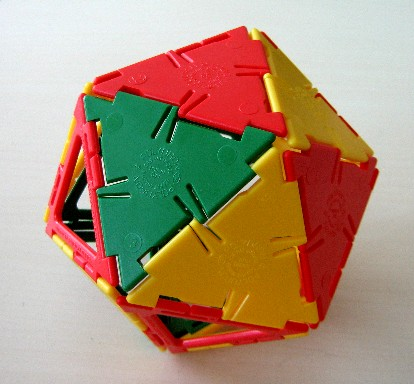degree (number of faces or edges) at each vertex 3 3 4 3 5 V=number of vertices 4 8 6 20 12 E=number of edges 6 12 12 30 30 F=number of faces 4 6 8 12 20 degree (number of edges) of each face 3 4 3 5 3

Historical Note: The platonic solids were important to the Greek and Renaissance mathematicians. Plato matched fire, earth, air, water with tetrahedron, cube, octahedron, icosahedron. Kepler tried to find a relation between the 5 known planets and the 5 platonic solids.

Since polyhedra are also graph, we know from the graph page:

Theorem: Twice the number of edges equals the sum of the degrees of all vertices in every polyhedron.

Therefore the number of edges doesn't have to be counted but can be computed from the other parameters. In particular, since the graphs of Platonic solids are r-regular, (all vertices have the same degree r) we know that 2E=rV for them, i.e. E=rV/2. For instance, for the cube we have 3·8/2 edges, for the icosahedron we have 5·12/2 edges.

We want to be able to compute the number of edges also for non-Platonic polyhedra, i.e. for nonregular graphs. For this purpose we use the abbreviation of Vn for the number of vertices of degree n in every polyhedron, and in the same way Fn for the number of faces that are n-gons (bounded by n edges). Note that no vertex can have degree 2 in a polyhedron (Why not?)(although in a general graph it can) and of course, there are no 2-gons. Therefore Vn and Fn are always both equal to 0. Of course, since every vertex and every face have exactly one degree, V = V3+V4+V5+... and F = F3+F4+F5+... .

Class Activity: For the polyhedra constructed, the students are supposed to count the number of faces (F), edges (E), and vertices (V). The students should also record what polygons (for example, triangles, pentagons, n-gons) were used and all occuring degrees in the polyhedron. All data for the different student groups is collected in a large table on the blackboard. The students are asked whether they see a pattern in the data..
 Name doghouse double pyramid Napoleon's hat UFO Look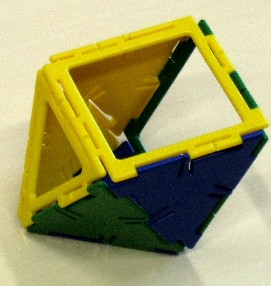The base is considered to be one 6-gon, instead of six triangles. V3,V4,V5.... 6,3 2,3 2,2,2 2,5 0,6,0,2 6,0,0,1 V=number of vertices 9 5 6 7 8 7 E=number of edges 15 = (3·6+4·3)/2 = (3·5+4·0+5·3)/2 9 = (3·2+4·3)/2 = 3·6/2 12 = (3·2+4·2+5·2)/2 = 3·8/2 13 = (3·2+4·5)/2 = (3·6+4·2)/2 18 = (3·0+4·6+5·0+6·2)/2 = 3·12/2 12 = (3·6+4·0+5·0+6·1)/2 =(3·6+4·0+5·0+6·1)/2 F=number of faces 8 6 8 8 12 7 F3,F4,F5,... 5,0,3 6 (all triangles) 8 (all triangles) 6,2 12 (all triangles) or: 6,0,0,1 nonconvex self-dual

Now the sum of all degrees of all vertices equals 3·V3 +4·V4+5·V5+ .... . Therefore the above remark can be restated as:

Corollary: In every polyhedron 2E = 3·V3+4·V4+5·V5+ .... .

## The Dual of a Polyhedron

Start with any polyhedron. The middle of each face will become a new vertex. Now connect those new vertices by straight lines---the new edges---provided the corresponding faces share an edge. We get another polyhedron, having as many edges as the old one, as many vertices as the old one had faces, and as many faces as the old one had vertices. The dual of the cube is the octahedron, and the dual of the dodecahedron is the icosahedron. The tetrahedron is its own dual. The dual of the dual is the old poyhedron.

Recall that the degree of a face is the number of edges bounding it. It equals the degree of the corresponding vertex in the dual.

Theorem: Twice the number of edges equals the sum of the degrees of all faces in every polyhedron.
Proof 1:Consider the dual of the polyhedron, where by the Theorem above 2E equlas the sum of all degrees of all verticves of the dual. But these vertices correspond to faces in the original polyhedron, and the degrees of corresponding vertices/faces are identical. the original polyhedron and its dual have also the same num ber of edges. Thus we get the equation above for the original polyhedron.
Proof 2:This result could also be derived directly, without using the dual, just by ordinary double counting.

Corollary: In every polyhedron 2E = 3·F3+4·F4+5·F5+ .... .

## Truncated Polyhedra

Truncating a polyhedron means: All vertices are sliced off (with a sharp knife). The cuts form new faces, with new vertices and new edges. Here you can see the truncated dodecahedron, and here the truncated icosahedron---the soccer ball, also displayed above in the logo.

How do V, E, F of the original polyhedron relate to vertex number VT, edge number ET, and face number FT of the truncated polyhedron?

• All former faces remain, although in modified form. Additionaly, at each former vertex a face from the cut pops up. Therefore FT=F+V.
• All former edges also remian, although shortened. Additionaly, at each cut at former vertex x, d(x) new edges pop up, where d(x) is the degree of that vertex. Therefore we get as many new edges as the sum of all former degrees. According to our previous formula, this number equals 2E. Therefore ET = E+2E=3E.
• All old vertices are cut off, but at every former vertex x, d(x) new vertices pop up at the cut face. therefore we get as many new vertices as the sum of all former degrees is, which again equals 2E. Therefore VT=2E.

## Glueing Polyhedra together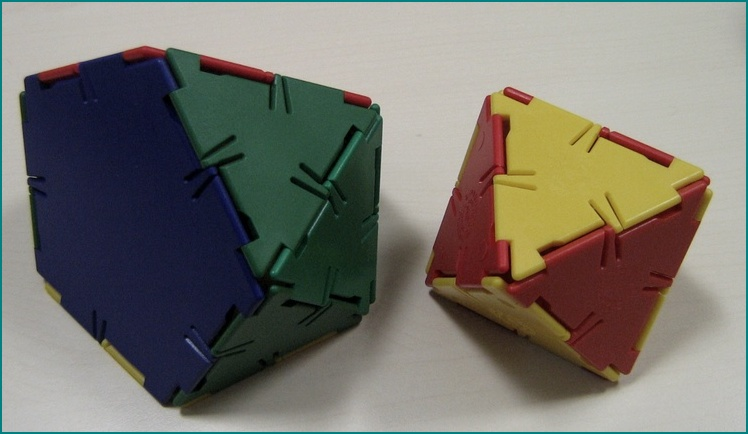... ...... ...

In the same way as above, let us investigate how the vertex number VG, edge number EG, and face number FG of the glued polyhedron relate to these parameters V1, E1, F1 of the first polyhedron and V2, E2, F2 of the second polyhedron. Assume both polyhedra are glued together along an n-gon.

• Two faces are lost in order to make the glueing possible, one from each former polyhedron. Therefore FG = F1-1+F2-1 = F1+F2-2
• All edges remain, but the n edges in each of the glued n-gon are identified. Thus overall n edges get lost by the process. We get EG = E1+E2-n.
• In the same way than with the edges, n vertices get lost too, since the vertices on the two n-gons are identified. Thus we get VG = V1+V2-n.

Our data proved that you cannot make a polyhedron with less edges than faces.